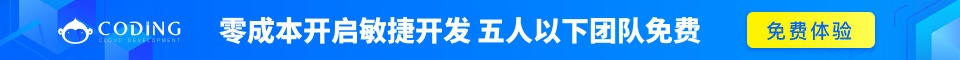# Java分支结构 – if…else/switch

Java有两种分支结构：

• if语句
• switch语句

## if语句

### 语法

If语句的用语法如下：

```if(布尔表达式)
{
//如果布尔表达式为true将执行的语句
}
```

```public class Test {

public static void main(String args[]){
int x = 10;

if( x < 20 ){
System.out.print("这是 if 语句");
}
}
}
```

```这是 if 语句
```

## if...else语句

if语句后面可以跟else语句，当if语句的布尔表达式值为false时，else语句块会被执行。

### 语法

if…else的用法如下：

```if(布尔表达式){
//如果布尔表达式的值为true
}else{
//如果布尔表达式的值为false
}
```

### 实例

```public class Test {

public static void main(String args[]){
int x = 30;

if( x < 20 ){
System.out.print("这是 if 语句");
}else{
System.out.print("这是 else 语句");
}
}
}
```

```这是 else 语句
```

## if...else if...else语句

if语句后面可以跟elseif…else语句，这种语句可以检测到多种可能的情况。

• if语句至多有1个else语句，else语句在所有的elseif语句之后。
• If语句可以有若干个elseif语句，它们必须在else语句之前。
• 一旦其中一个else if语句检测为true，其他的else if以及else语句都将跳过执行。

### 语法

if...else语法格式如下:

```if(布尔表达式 1){
//如果布尔表达式 1的值为true执行代码
}else if(布尔表达式 2){
//如果布尔表达式 2的值为true执行代码
}else if(布尔表达式 3){
//如果布尔表达式 3的值为true执行代码
}else {
//如果以上布尔表达式都不为true执行代码
}
```

### 实例

```public class Test {

public static void main(String args[]){
int x = 30;

if( x == 10 ){
System.out.print("Value of X is 10");
}else if( x == 20 ){
System.out.print("Value of X is 20");
}else if( x == 30 ){
System.out.print("Value of X is 30");
}else{
System.out.print("This is else statement");
}
}
}
```

```Value of X is 30
```

## 嵌套的if…else语句

### 语法

```if(布尔表达式 1){
////如果布尔表达式 1的值为true执行代码
if(布尔表达式 2){
////如果布尔表达式 2的值为true执行代码
}
}
```

### 实例

```public class Test {

public static void main(String args[]){
int x = 30;
int y = 10;

if( x == 30 ){
if( y == 10 ){
System.out.print("X = 30 and Y = 10");
}
}
}
}
```

```X = 30 and Y = 10
```

## switch语句

switch语句判断一个变量与一系列值中某个值是否相等，每个值称为一个分支。

### 语法

switch语法格式如下：

```switch(expression){
case value :
//语句
break; //可选
case value :
//语句
break; //可选
//你可以有任意数量的case语句
default : //可选
//语句
}
```

switch语句有如下规则：

• switch语句中的变量类型只能为byte、short、int或者char。
• switch语句可以拥有多个case语句。每个case后面跟一个要比较的值和冒号。
• case语句中的值的数据类型必须与变量的数据类型相同，而且只能是常量或者字面常量。
• 当变量的值与case语句的值相等时，那么case语句之后的语句开始执行，直到break语句出现才会跳出switch语句。3
• 当遇到break语句时，switch语句终止。程序跳转到switch语句后面的语句执行。case语句不必须要包含break语句。如果没有break语句出现，程序会继续执行下一条case语句，直到出现break语句。
• switch语句可以包含一个default分支，该分支必须是switch语句的最后一个分支。default在没有case语句的值和变量值相等的时候执行。default分支不需要break语句。

### 实例

```public class Test {

public static void main(String args[]){

{
case 'A' :
System.out.println("Excellent!");
break;
case 'B' :
case 'C' :
System.out.println("Well done");
break;
case 'D' :
System.out.println("You passed");
case 'F' :
System.out.println("Better try again");
break;
default :
```\$ java Test